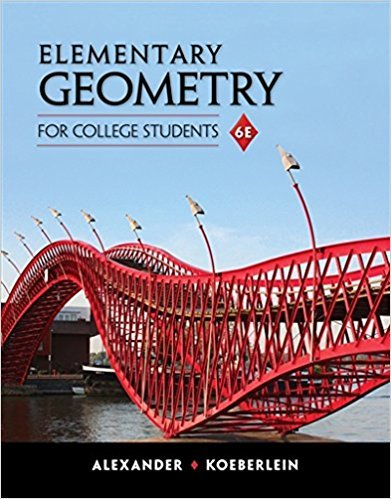×
×

# Solutions for Chapter 9: Surfaces and Solids## Full solutions for Elementary Geometry for College Students | 6th Edition

ISBN: 9781285195698Solutions for Chapter 9: Surfaces and Solids

Solutions for Chapter 9
4 5 0 334 Reviews
14
0
##### ISBN: 9781285195698

Since 50 problems in chapter 9: Surfaces and Solids have been answered, more than 4233 students have viewed full step-by-step solutions from this chapter. Elementary Geometry for College Students was written by and is associated to the ISBN: 9781285195698. This expansive textbook survival guide covers the following chapters and their solutions. This textbook survival guide was created for the textbook: Elementary Geometry for College Students, edition: 6. Chapter 9: Surfaces and Solids includes 50 full step-by-step solutions.

Key Math Terms and definitions covered in this textbook
• Column space C (A) =

space of all combinations of the columns of A.

• Covariance matrix:E.

When random variables Xi have mean = average value = 0, their covariances "'£ ij are the averages of XiX j. With means Xi, the matrix :E = mean of (x - x) (x - x) T is positive (semi)definite; :E is diagonal if the Xi are independent.

• Full row rank r = m.

Independent rows, at least one solution to Ax = b, column space is all of Rm. Full rank means full column rank or full row rank.

• Fundamental Theorem.

The nullspace N (A) and row space C (AT) are orthogonal complements in Rn(perpendicular from Ax = 0 with dimensions rand n - r). Applied to AT, the column space C(A) is the orthogonal complement of N(AT) in Rm.

• Identity matrix I (or In).

Diagonal entries = 1, off-diagonal entries = 0.

• Iterative method.

A sequence of steps intended to approach the desired solution.

• lA-II = l/lAI and IATI = IAI.

The big formula for det(A) has a sum of n! terms, the cofactor formula uses determinants of size n - 1, volume of box = I det( A) I.

• Multiplier eij.

The pivot row j is multiplied by eij and subtracted from row i to eliminate the i, j entry: eij = (entry to eliminate) / (jth pivot).

• Normal equation AT Ax = ATb.

Gives the least squares solution to Ax = b if A has full rank n (independent columns). The equation says that (columns of A)·(b - Ax) = o.

• Nullspace N (A)

= All solutions to Ax = O. Dimension n - r = (# columns) - rank.

• Outer product uv T

= column times row = rank one matrix.

• Plane (or hyperplane) in Rn.

Vectors x with aT x = O. Plane is perpendicular to a =1= O.

• Polar decomposition A = Q H.

Orthogonal Q times positive (semi)definite H.

• Random matrix rand(n) or randn(n).

MATLAB creates a matrix with random entries, uniformly distributed on [0 1] for rand and standard normal distribution for randn.

• Rayleigh quotient q (x) = X T Ax I x T x for symmetric A: Amin < q (x) < Amax.

Those extremes are reached at the eigenvectors x for Amin(A) and Amax(A).

• Semidefinite matrix A.

(Positive) semidefinite: all x T Ax > 0, all A > 0; A = any RT R.

• Solvable system Ax = b.

The right side b is in the column space of A.

• Standard basis for Rn.

Columns of n by n identity matrix (written i ,j ,k in R3).

• Trace of A

= sum of diagonal entries = sum of eigenvalues of A. Tr AB = Tr BA.

• Transpose matrix AT.

Entries AL = Ajj. AT is n by In, AT A is square, symmetric, positive semidefinite. The transposes of AB and A-I are BT AT and (AT)-I.

×